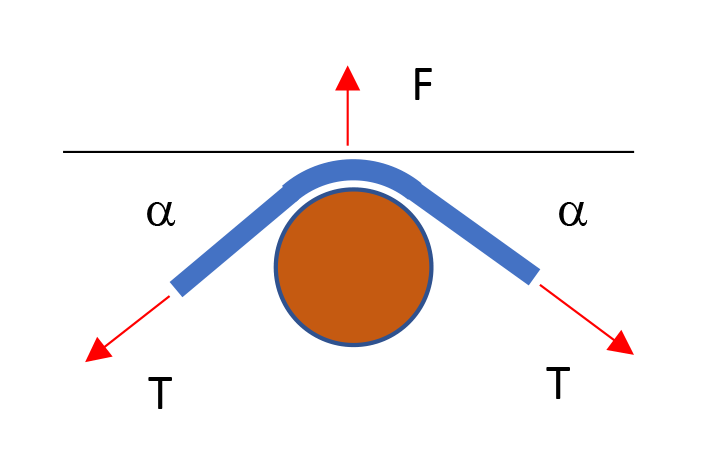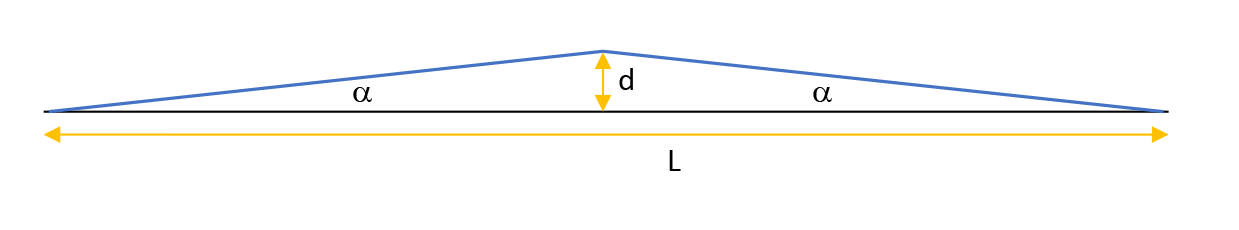# All String Equations in One PlaceI gave my students the job of building string instruments, for which they need to select the materials, calculate the appropriate string gauges and tensions, and pay attention to the energy flow from strings to air. After spending half a day putting the relevant equations in one sheet to make their work easier, it dawned on me that others might enjoy having them as well. So here they are.

String equation:

This relates the fundamental frequency of a vibrating string, f, to its tension T, length L, and physical properties, which reduces to the linear density μ, or mass per unit length. There is a pretty good Wikipedia article on this, leading to this equation:

f = 1//2L √(T/µ)

In turn, the linear density µ is a function of the string diameter D and the bulk density ρ though this equation, which assumes the string to be a long cylinder:

µ = π D2 ρ/4

If you use D’Addario strings, they have put together a handy reference on many of their strings, including linear densities and tensions.

Here are some (pretty hard to find) bulk densities, in g/cm3,  for common string materials:

 Nylon Nylgut (ukulele) Fluorocarbon Classical wound Steel Nickel wound (electric) Bronze wound (acoustic) 1.076 1.325 1.812 4.915 7.726 6.533 7.073

And here are some common frequencies, for the 4th octave in “concert 440” standard pitch:

 Note C4 D4 E4 F4 G4 A4 B4 Freq. (Hz) 261.62 293.66 329.6 349.2 392 440 493.9

Up an octave: multiply frequency by 2. Down an octave: divide frequency by 2.

Fret equation:

There is a decent Wikipedia article on this, but still you are likely to find a lot of Internet sources telling you about the “18 rule” to place the first fret: divide the remaining length between a fret and the bridge by 18, and this is the distance to the next fret. This is nonsense for two reasons:

1. The actual factor is an irrational number, which is about 17.817. It doesn’t look like a lot, but the error is considerable and will cause your instrument to be badly intoned after only a few frets.
2. If you add each fret based on the position of the previous fret, you’ll be accumulating the error incurred in calculating (or simply marking) each fret position. It is best to use a constant reference for all fret positions so errors don’t accumulate.

So here’s the better (and simpler) fret equation:

r = (1/2)1/12 = 0.943873…

where r is the constant factor by which the distance from bridge to fret shrinks with each successive fret. Apply it to the scale length to find the distance from bridge to fret 1, and so on. It also happens to be the factor by which the distance between successive frets shrinks, but don’t use it this way because you’d end up accumulating errors.

Tension calculator:In this other article, I describe a simple way to measure string tension, which you will need for the string equation. But here’s the tension equation again:

T = (FL)/(4d)

where F is the reading of a force scale pulling the string sideways at the midpoint (12th fret), L the scale length, and d the displacement at the middle of the string when you do this.This site uses Akismet to reduce spam. Learn how your comment data is processed.